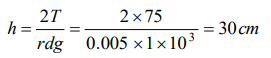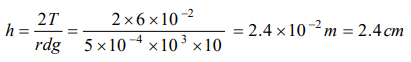## Mechanical Properties of Fluids Questions and Answers Part-15

1. A vessel, whose bottom has round holes withdiameter of 0.1mm, is filled with water. The maximum height to which the water can be filled without leakage is $\left(S.T. of water =75 dyne/cm,g =1000 cm\diagup s^{2}\right)$
a) 100 cm
b) 75 cm
c) 50 cm
d) 30 cm

Explanation:2.Water rises in a capillary tube when its one end is dipped vertically in it, is 3 cm. If the surface tension of water is $75\times 10^{-3} N\diagup m$    , then the diameter of capillary will be
a) 0.1 mm
b) 0.5 mm
c) 1.0 mm
d) 2.0 mm

Explanation:3. A capillary tube made of glass is dipped into mercury. Then
a) Mercury rises in the capillary tube
b) Mercury rises and flows out of the capillary tube
c) Mercury descends in the capillary tube
d) Mercury neither rises nor descends in the capillary tube

Explanation: The angle of contact of mercury with glass is obtuse. So it gets depressed below the liquid level outside

4. By inserting a capillary tube upto a depth l in water, the water rises to a height h. If the lower end of the capillary is closed inside water and the capillary is taken out and closed end opened, to what height the water will remain in the tube
a) Zero
b) $l+h$
c) 2h
d) h

Explanation: The water rises to height h due to capilarity

5. If the diameter of a capillary tube is doubled, then the height of the liquid that will rise is
a) Twice
b) Half
c) Same as earlier
d) None of these

Explanation:6. If the surface tension of water is 0.06 Nm-1, then the capillary rise in a tube of diameter 1 mm is $\left(\theta =0^{\circ}\right)$
a) 1.22 cm
b) 2.44 cm
c) 3.12 cm
d) 3.86 cm

Explanation:7. Two capillary tubes of radii 0.2 cm and 0.4 cm are dipped in the same liquid. The ratio of heights through which liquid will rise in the tubes is
a) 1 : 2
b) 2 : 1
c) 1 : 4
d) 4 : 1

Explanation:8. A capillary tube when immersed vertically in liquid records a rise of 3 cm. If the tube is immersed in the liquid at an angle of 600 with the vertical. The length of the liquid column along the tube is
a) 9cm
b) 6cm
c) 3cm
d) 2cm

Explanation:9. The action of a nib split at the top is explained by
a) Gravity flow
b) Diffusion of fluid
c) Capillary action
d) Osmosis of liquid

a) $r=\frac{2T\cos\theta}{hdg}$
b) $r=\frac{hdg}{2T\cos\theta}$
c) $r=\frac{2Tdgh}{\cos\theta}$
d) $r=\frac{T\cos\theta}{2hdg}$
Explanation: $r=\frac{2T\cos\theta}{hdg}$